# Dijkstra’s Shortest Path Algorithm

The Dijkstra’s Shortest Path algorithm is a greedy algorithm which is used for finding the shortest path between nodes in a graph. This algorithm finds the shortest path between the two nodes but it can be used for finding the shortest paths from a single node to all other nodes by iterating the algorithm for more than once (Total number of nodes – 1).

## Working of Dijkstra’s Shortest Path algorithm

Dijkstra’s Shortest Path algorithm is working on the greedy approach. In this shortest path tree with given source as a root is generated. And every step of the algorithm finds the vertex has a minimum distance from the source.
It uses the property in the opposite direction that if the shortest path between vertices A and C that is A -> C and B -> C is the subpath in A -> C then B -> C is also the shortest path between vertices B and C.

## Example of Dijkstra’s algorithm

Greedy algorithm approach is used for finding the shortest path between nodes in a graph.
Below is the example of Dijkstra’s algorithm with input- output constraint and the solution for the example.

### Input and Output of the Example

Given the weighted graph G, and the task is used to find the shortest path between nodes.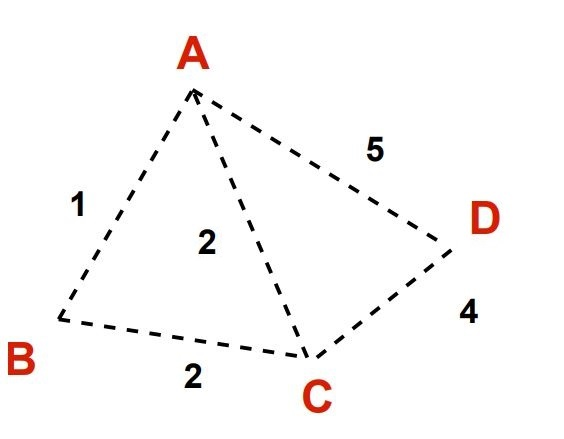Solution: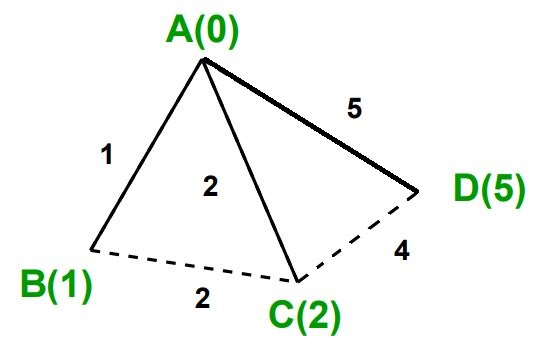### Stepwise Solution of the Problem Example using Dijkstra’s Shortest Path Algorithm

Step 1: Start with the given weighted graphStep 2: Pick the starting vertex and assign infinity path values to all other vertices.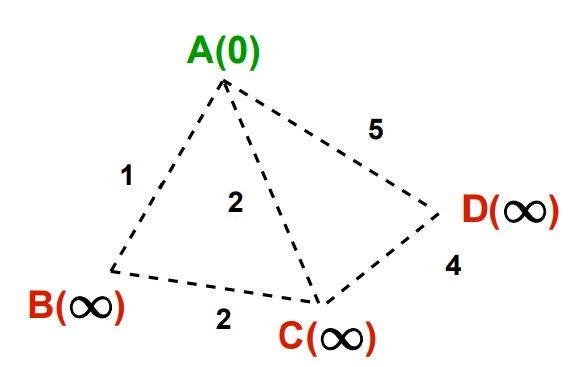Step 3: Go to each vertex adjacent to previous vertex and update its path length.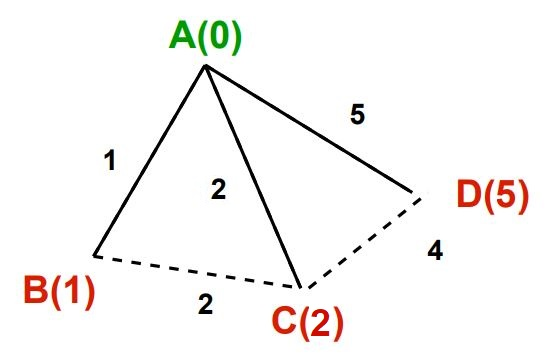Step 4: If the path length of adjacent vertex is less than new path don’t update it and avoid updating path lengths of already visited vertices.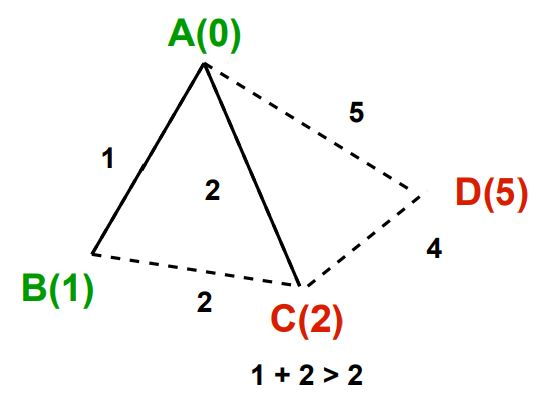Step 5: After each iteration, pick unvisited with least path and repeat until all vertices have been visited.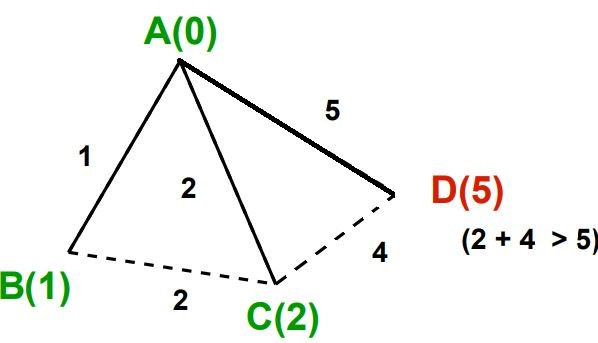Step 6: Return the solution## Pseudocode for Dijkstra’s Shortest Path algorithm

Here’s Pseudocode for finding the shortest path between the nodes in a graph using greedy algorithm approach.

``````Dijkstra(Graph, source):
// Initializations
for each vertex v in Graph:
// Unknown distance function from source to
//each node set to infinity
dist[v]  <- infinity

// All nodes initially in Q
add v to Q

// Distance from source to source is set to 0
dist[source]  := 0

while Q is not empty:
v <- vertex  min dist[v] from Q

remove v from Q

for each neighbor u of v:
alt := dist[v] + length(v, u)

// A shorter path to u has been found
if alt < dist[u]:

// Update distance of u
dist[u]  := alt
return dist[]
``````

## Implementation of Dijkstra’s Shortest Path Algorithm in Python

``````# Dijkstra's Algorithm in Python
import sys

# Function to find which vertex is to be visited next
def next_to_visited():
global visited
v = -10
for index in range(num_of_vertices):
if visited[index] == 0 and (v < 0 or visited[index] <= visited[v]):
v = index
return v

# Function to find the shortest path between nodes in a graph
def dijkstra():
for vertex in range(num_of_vertices):

# Find next vertex to be visited
visit = next_to_visited()

for index in range(num_of_vertices):

# Updating new distances
if vertices[visit][index] == 1 and visited[index] == 0:
new_distance = visited[visit] + edges[visit][index]
if visited[index] > new_distance:
visited[index] = new_distance

visited[visit] = 1

# Printing the distance
def print_dist():
i = 0
for distance in visited[1:]:
print("Distance from S ->", chr(ord('A')+i), ":",distance)
i = i + 1

# Driver Code
# Providing the graph
# 2D array to represnt the vertex structure
vertices = [[0, 1, 1, 1],
[1, 0, 1, 0],
[1, 1, 0, 1],
[1, 0, 1, 0]]

# 2D array to represnt the edge structure
edges = [[0, 1, 2, 5],
[1, 0, 2, 0],
[2, 2, 0, 4],
[5, 0, 4, 0]]

# calculate Number of vertices
num_of_vertices = len(vertices)

# store the visited edge and vertex
visited = [[0, 0]]
for i in range(num_of_vertices-1):
visited.append([0, sys.maxsize])

# Function calling
dijkstra()
print_dist()
``````
Scroll to Top
[gravityforms id="5" description="false" titla="false" ajax="true"]